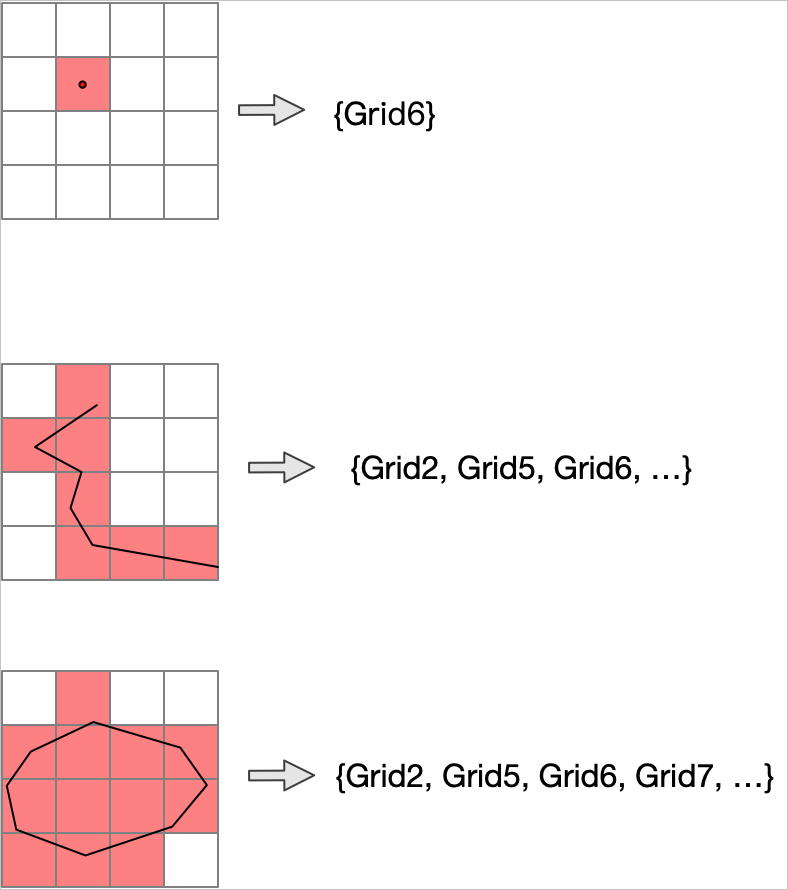This topic describes the ST_AsGrid function, which queries the grids that intersect with a geometry.

## Syntax

``geomgrid[] ST_AsGrid(geometry geom, integer precision);``

## Parameters

Parameter Description
geom The geometry that you want to query.
precision The precision level based on which you want to query the geometry. Valid values: 0 to 31.

## Description

The geometry must use the CGC2000 spatial reference system. In addition, the spatial reference system identifier (SRID) of the geometry must be 4490. If the geometry does not use the CGC2000 spatial reference system, the ST_Transform function is invoked to convert the coordinates of the geometry into CGC2000 coordinates.

This function returns an array that consists of geometry-represented grids that intersect. The following figures are examples of how grids intersect with a point, a line, and a polygon.## Examples

``````select st_astext(st_asgrid(
ST_geomfromtext('POINT(116.31522216796875 39.910277777777778)',4490), 15))

st_astext
--------------------
{G001310322230230}

select st_astext(st_asgrid(
ST_geomfromtext('LINESTRING(122.48077 51.72814,122.47416 51.73714)',4490), 18))

st_astext
--------------------------------------------------------------------------------
{G001331032213300011,G001331032213300013,G001331032213122320,G00133103221312232
2,G001331032213300100,G001331032213122303,G001331032213122321,G00133103221312231
2}``````